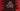# Java Program to convert an ArrayList to an Array### Introduction :

ArrayList is more flexible than the array. But sometimes we need to convert an ArrayList to an array and this tutorial will show you different ways to convert ArrayList to an array in Java. We have different ways to do this conversion. Let’s take a look :

### Using a loop :

This is the most simple way to solve this problem. First, create one empty array. Use one loop, iterate through the ArrayList and put each ArrayList element in the array. The main thing is that you will have to create an array of the same size as the ArrayList.

``````import java.util.ArrayList;
import java.util.Arrays;

public class Main {
public static void main(String[] args) {
//1
ArrayList days = new ArrayList<>();

//2

//3
String[] daysArray = new String[days.size()];

//4
for (int i = 0; i < days.size(); i++) {
daysArray[i] = days.get(i);
}

//5
System.out.println("Final array : " + Arrays.toString(daysArray));
}
}``````

### Explanation :

The commented numbers in the above program denote the step number below :

1. First of all, create one ArrayList days. We will store the day of the week in this list.
2. Add the string name of each day to the ArrayList days.
3. Now, we need to create one Array that holds all the values of this ArrayList. First of all, create one String array object with the same size as days. The name of the object is daysArray.
4. Run one for loop to iterate through each element of days. Insert the value of each element to the string array. Since the size of the array is the same as the ArrayList, it will not cause any problem.
5. Finally, print out the array. Convert the array to a string using toString(), or else we will not be able to see the values.

It will print the below output :

``Final array : [sunday, monday, tuesday, wednesday, thrusday, friday, saturday]``

### Using toArray() :

We can also convert ArrayList to an array using toArray() method. So, by using days.toArray(); in the above example, we will get one array of all the elements of this ArrayList. But the problem is that toArray() returns an array of Object not an array of String. So, if we try to convert it like String[] daysArray = days.toArray();, it will throw one exception.

We can use one different method toArray(T[] a) to get an array of Strings. This method takes one array of any type as the parameter. It will store the elements of the ArrayList in this array. If the array size is small, it will create one array of similar type and store the elements in that array. Finally, it will return us the array holding all elements. In our case, we will pass one string array of size 0 to this method. It will create one array of the same size as the ArrayList and return us back.

``````import java.util.ArrayList;
import java.util.Arrays;

public class Main {
public static void main(String[] args) {
ArrayList days = new ArrayList<>();

String[] daysArray = days.toArray(new String);

System.out.println("Final array : " + Arrays.toString(daysArray));
}
}``````

The output is same as above :

``Final array : [sunday, monday, tuesday, wednesday, thrusday, friday, saturday]``

### Using Java 8 Stream :

Stream in Java-8 provides a lot of useful functions. Using stream, we can also convert ArrayList to an array.

``````import java.util.ArrayList;
import java.util.Arrays;

public class Main {
public static void main(String[] args) {
ArrayList days = new ArrayList<>();